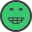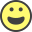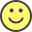Mathematical terms: Inglês para matemática

Here I will provide some equations, and then write how to read them.

3 + 7 = 10
Three plus seven equals ten.
Three plus seven is equal to ten.

y = 3/8
Y equals three divided by eight.
Y equals three over eight.
Y equals three eighths.

f(x) = x²
F of X equals X squared

f(x) = (x + 5)²
F of X equals X plus five quantity squared.

log 1000 = 3
Log of one thousand equals three.

ln 0 = 1
Natural log of zero equals 1.

For f(x)=x², the domain of f = ∀x | x∈R
For F of X equals X squared, the domain of f equals all (values of) X such that x is an element of R. OR

For f(x)=x², domain = R
For F of X equals X squared, the domain is all real numbers

lim x->2 (5x)/(x + 3) = 2
The limit as X approaches two, of five X over X plus three, equals two. OR
The limit of five X over X plus three, as X approaches two, equals two.

f(x) = x² | x<0
F of X equals X squared, such that X is less than zero.

C = 2πr
Circumference is equal to two pi R.

∫ f(x) dx
The indefinite integral of F of X D X. OR
The indefinite integral of F of X with respect to X.

y' = 3x²
Y prime equals three X squared.

(cosx)' = -sinx
Cosine X prime equals negative sine X.

d/dx tanˉ¹(x) = [tanˉ¹(x)]' = 1/(x² + 1)
D DX inverse tan X is equal to inverse tan X prime which is equal to one over X squared plus one.

f( g(x) ) = ...
F of G of X equals ...

Feel free to add more or add one that you would like to learn... Or if you need math help, I can try to help too.ATIVE O ENGLISH PLUS POR R\$ 8/MÊS Além de aprender sem anúncios, você terá acesso: aos Cursos do English Experts, a respostas verificadas por especialistas (ilimitado) e ao aplicativo Meu Vocabulário.
10 respostas
1 2
These are very useful. Thanks for sharing.
(online) 3 11 91
Só para complementar. Publiquei um post sobre o tema no blog faz algum tempo

https://www.englishexperts.com.br/expre ... em-ingles/

See you!
Thanks!Hello guys!

I've been studying how to name math expressions, and I've found something like:

(a + b + c + d) / e

What's the best/right way to say it?
1- a plus b, plus c, plus d, all over e
or
2- a plus b, plus c, plus d, over e

Thank you!3 18 183
mhenrique escreveu:Hello guys!

I've been studying how to name math expressions, and I've found something like:

(a + b + c + d) / e

What's the best/right way to say it?
1- a plus b, plus c, plus d, all over e
or
2- a plus b, plus c, plus d, over e

Thank you!Normalmente, a primeira deixa mais claro o pretendido. A omissão de "all over" ocorreria se alguém estivesse escrevendo a expressão ao mesmo tempo, no quadro-negro, por exemplo.

Oralmente, outra opção seria: "Bracket, a+b+c+d, close bracket, over e."
Huum... I got it!

Thanks man, you helped me so muchAproveitando o tópico. Como eu diria : Escreva as operações (matemáticas) . O contexto é : o professor vai ditar as "continhas" em ingles para os alunos dele. Então ele quer que eles escrevam a instrução da atividade em ingles.

Escreva as operações/"continhas" em ingles.

Usa math expressions também?

Agradecida9 62 593
dirci perguntou:
Aproveitando o tópico. Como eu diria : Escreva as operações (matemáticas) . O contexto é : o professor vai ditar as "continhas" em ingles para os alunos dele. Então ele quer que eles escrevam a instrução da atividade em ingles.

Escreva as operações/"continhas" em ingles.
Write down the mathematical operations.
2 16 113
How can I say ''Progressão Aritmética' e 'Função quadrática '?
6 48 1.1k
Progressão Aritmética = Arithmetic Progression, that is no more than an aritmetic sequence. The latter, a list of numbers where each number is equal to the previous number, plus a constant (called common reference - in Portuguese "razão").
It´s considered as "general series" at WolframMathWorld (a site dedicated to Maths, languages, codes etc.)

http://www.mathportal.org/calculators/s ... ulator.php
http://mathworld.wolfram.com/topics/GeneralSeries.html

Função Quadrática - Quadratic Function. "Is a function or mathematical expression of degree two. This means that the highest power of the function is two."
Its graph is parabola-shaped. That is, aproximately U-shapped (an mirror-symmetrical curve).